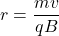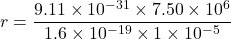## A cosmic ray electron moves at 7.50×106 m/s perpendicular to the Earth’s magnetic field at an altitude where field strength is 1.00×10−5 T .

Question

A cosmic ray electron moves at 7.50×106 m/s perpendicular to the Earth’s magnetic field at an altitude where field strength is 1.00×10−5 T . What is the radius of the circular path the electron follows?

in progress 0
5 months 2021-08-15T00:07:05+00:00 1 Answers 105 views 0

4.266 m.

Explanation:

Given,

Speed of the electron = 7.50 x 10⁶ m/s

Magnetic field, B = 1 x 10⁻⁵ T

radius of circular path = ?

Using formula of radius of curvature of the charge particlem is mass of electron

q is charge of electronr = 4.266 m

Hence, radius of circular path is equal to 4.266 m.